Courses

# Test: Geometry

## 30 Questions MCQ Test Topic-wise Past Year Questions for CAT | Test: Geometry

Description
This mock test of Test: Geometry for CAT helps you for every CAT entrance exam. This contains 30 Multiple Choice Questions for CAT Test: Geometry (mcq) to study with solutions a complete question bank. The solved questions answers in this Test: Geometry quiz give you a good mix of easy questions and tough questions. CAT students definitely take this Test: Geometry exercise for a better result in the exam. You can find other Test: Geometry extra questions, long questions & short questions for CAT on EduRev as well by searching above.
QUESTION: 1

### Let S be the set of all points (x, y) in the x-y plane such that |x| + |y| ≤ 2 and |x| ≥ 1. Then, the area (in square units) of the region represented by S equals (2019)

Solution:

The below figure shows the graph of both the given expressions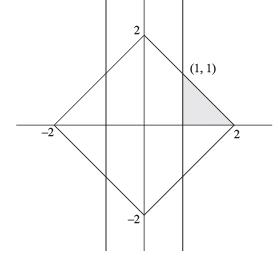The required area = 4 × the area of the shaded triangle.
Area of the shaded triangle =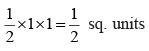Therefore, the required area =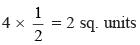QUESTION: 2

### AB is a diameter of a circle of radius 5 cm. Let P and Q be two points on the circle so that the length of PB is 6 cm, and the length of AP is twice that of AQ. Then the length, in cm, of QB is nearest to (2019)

Solution: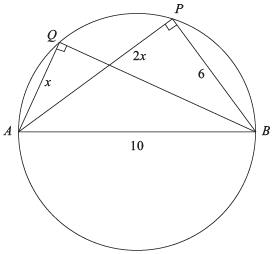Since AB  is a diameter, therefore ∠AQB and ∠APB are angles in the semi–circle and hence are right–angles.
i.e. ∠A PB = ∠AQB = 90°
Let AQ  = x, then AP = 2x
In right ΔAPB,
AP2 = AB2 – BP2 ⇒ AP2 = 102 – 62 = 82
⇒ AP = 8
⇒ 2x = 8
⇒ x = 4
In right ΔAQB
BQ2 = AB2 – AQ2
⇒ BQ2 = 102 – 42 = 84
⇒ BQ = √84 ≈ 9.1

QUESTION: 3

### With rectangular axes of coordinates, the number of paths from (1, 1) to (8, 10) via (4, 6), where each step from any point (x, y) is either to (x, y + 1) or to (x + 1, y), is (2019)

Solution:

Let A, B  and C represent the coordinates (1, 1), (8, 10) and (4, 6) respectively.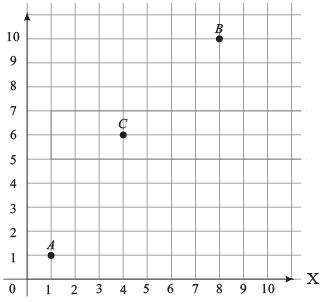The number of ways to go from A to B via C
= (The number of ways to go from A to C) × (The number of ways to go from C to B)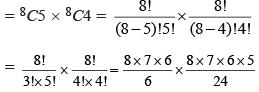56 × 70 = 3920

QUESTION: 4

Let T be the triangle formed by the straight line 3x + 5y – 45 = 0 and the coordinate axes. Let the circumcircle of T have radius of length L, measured in the same unit as the coordinate axes. Then, the integer closest to L is

(2019)

Solution:

Clearly, the triangle will be right angled triangle and its hypotenuse would be the diameter of its circumcircle.
Given equation, 3x + 5y – 45 = 0
When y = 0, Then x = 15
When x = 0, Then y = 9
Hence, A(15, 0) and B(0, 9) and O(0, 0) are the vertices of the right triangle formed whose hypotonuse is the diameter of the ΔOAB.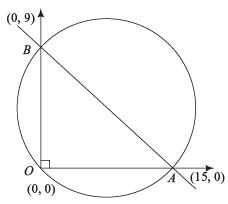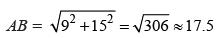∴ Now, Radius(L) =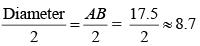Hence, the integer closest to L = 9.

QUESTION: 5

In a circle of radius 11 cm, CD is a diameter and AB is a chord of length 20.5 cm. If AB and CD intersect at a point E inside the circle and CE has length 7 cm, then the difference of the lengths of BE and AE, in cm, is

(2019)

Solution: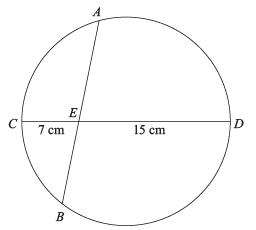In the figure, AB and CD are two chords of a circle, intersects each other at point E.
∴ AE × BE = CE × DE
⇒ AE × BE = 7 × 15 = 105
(AE – BE)2 = (AE + BE)2 – 4 × AE × BE = (AB)2 – 4
× 105
⇒ (AE – BE)2 = (20.5)2 – 420
⇒ (AE – BE)2 = 420.25 – 420 = 0.25
⇒ AE – BE = 0.5

QUESTION: 6

Let ABC be a right-angled triangle with hypotenuse BC of length 20 cm. If AP is perpendicular on BC, then the maximum possible length of AP, in cm, is

(2019)

Solution: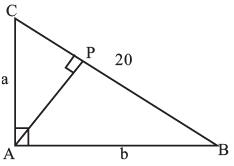In ΔABC, let AC = a and AB = b
Then by pythagoras theorem for ΔABC,
a2 + b2 = 202 = 400 ...(i)
In ΔABC and ΔPAC,
∠BAC = ∠APC (= 90°)
∠C = ∠C (common)
∴ ΔABC ~ ΔPAC (by AA)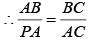[Ratio of corresponding sides of two similar triangles are equal]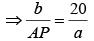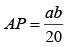...(ii)
For maximum value of AP, we have to maximize the product ab.
Applying AM ≥ GM inequality, we get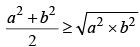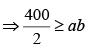⇒ ab ≤ 200
Hence, the maximum value of ab = 200
From eq. (ii), it is clear that value of AP will be maximum when value of ab will be maximum
∴ maximum value of AP = 200 / 20 = 10

QUESTION: 7

In a triangle ABC, medians AD and BE are perpendicular to each other, and have lengths 12 cm and 9 cm, respectively. Then, the area of triangle ABC, in sq cm, is

(2019)

Solution: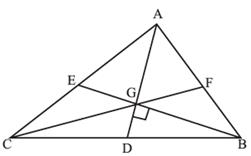Draw the third median CF. We know the following facts.
(i) The intersection point of medians i.e. centroid (G) divides each median into 2:1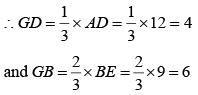(ii) All three medians divide the triangle into 6 parts of equal area.
Now, Area of triangle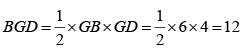∴ area of triangle ABC = 6 × (Area of ΔBGD)
= 6 × 12 = 72

QUESTION: 8

Two circles, each of radius 4 cm, touch externally. Each of these two circles is touched externally by a third circle. If these three circles have a common tangent then the radius of the third circle, in cm, is

(2019)

Solution: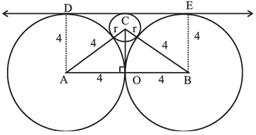From the figure, OC = AD – r = 4 – r
Applying Pythagoras theorem in right triangle AOC, we get
AC2 = AO2 + OC2
⇒ (4 + r)2 = 42 + (4 – r)2
⇒ (4 + r)2 – (4 – r)2 = 16
⇒ 8 × 2r = 16
∴ r = 1

QUESTION: 9

The base of a regular pyramid is a square and each of the other four sides is an equilateral triangle, length of each side being 20 cm. The vertical height of the pyramid, in cm, is

(2019)

Solution: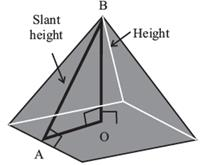From the diagram, it is clear that AB is the slant height of the equilateral triangle and is also the slant height of the pyramid.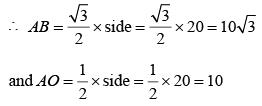On applying Pythagoras theorem in triangle AOB,
OB2 = AB2 – OA2 = (10√3 )2 − 102 = 200
∴ Height of the pyramid OB = 10√2 cm

QUESTION: 10

A man makes complete use of 405 cc of iron, 783 cc of aluminium, and 351 cc of copper to make a number of solid right circular cylinders of each type of metal. These cylinders have the same volume and each of these has radius 3 cm. If the total number of cylinders is to be kept at a minimum, then the total surface area of all these cylinders, in sq cm, is

(2019)

Solution:

To find the minimum number of cylinders, the volume of each of the cylinder must be HCF of 405, 783 and 351
HCF (405, 783, 351) = 27
Therefore, volume of each cylinder = 27cc
Hence number of cylinders of iron = 405 / 27 = 15
Number of cylinders of aluminium = 783 / 27 = 29
and number of cylinders of copper = 351 / 27 = 13
Therefore, the total number of a cylinders
= 15 + 29 + 13 = 57
Since, volume of each cylinder = 27cc
∴ πr2h = 27 ⇒ π × 32 × h = 27
⇒ h = 3 / π
Now, total surface area of each cylinder = 2πr (r + h)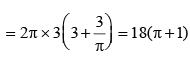∴ Total surface area of 57 cylinders
= 57 × 18(π + 1) sq. cm. = 1026 (π + 1)

QUESTION: 11

Let A and B be two regular polygons having a and b sides, respectively. If b = 2a and each interior angle of B is 3/2 times each interior angle of A, then each interior angle, in degrees, of a regular polygon with a + b sides is

(2019)

Solution:

The formula for each interior angle =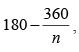where ‘n’
is the side of the regular polygon.
Now, (an interior angle of B) =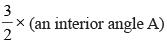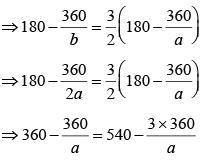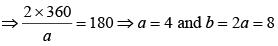Hence, polygon with each side = a + b = 4 + 8 = 12, will have each interior angle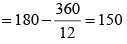QUESTION: 12

Points E, F, G, H lie on the sides AB, BC, CD, and DA, respectively, of a square ABCD. If EFGH is also a square whose area is 62.5% of that of ABCD and CG is longer than EB, then the ratio of length of EB to that of CG is

(2018)

Solution:

Let the area of ABCD be 100.
∴ Length of each side of ABCD = 10
Area of EFGH is 62.5,
Therefore length of each side of EFGH = √62.5
Triangles AEH, BFE, CGF and DHG are congruent by ASA.
Let AE = BF = CG = DH = x, then EB = FC = DG = AH = 10 – x
In right triangle EAH, AE2 + AH2 = EH2
x2 + (10 − x)2 = (√62.5)2 ⇒ 4x2 – 40x + 75 = 0
⇒ (2x – 5) (2x – 15) = 0
∴ x = 2.5 or 7.5
Since it’s given that CG is longer than EB, therefore CG = 7.5 and EB = 2.5.
∴ EB : CG = 1 : 3

QUESTION: 13

In a circle with center O and radius 1 cm, an arc AB makes an angle 60 degrees at O. Let R be the region bounded by the radii OA, OB and the arc AB. If C and D are two points on OA and OB, respectively, such that OC = OD and the area of triangle OCD is half that of R, then the length of OC, in cm, is

(2018)

Solution:

Area of ∆ OCD =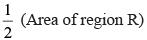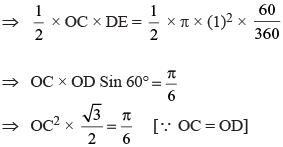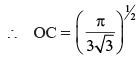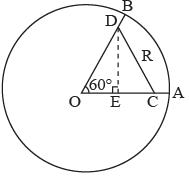QUESTION: 14

In a parallelogram ABCD of area 72 sq cm, the sides CD and AD have lengths 9 cm and 16 cm, respectively. Let P be a point on CD such that AP is perpendicular to CD. Then the area, in sq cm, of triangle APD is

(2018)

Solution:

Area of the parallelogram ABCD = (base) (height)
= CD × AP = 72 sq.cm.
⇒ 72 = 9 × (AP) ⇒ AP = 8cm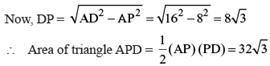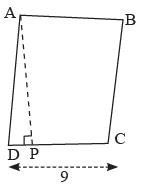QUESTION: 15

In a circle, two parallel chords on the same side of a diameter have lengths 4 cm and 6 cm. If the distance between these chords is 1 cm, then the radius of the circle, in cm, is

(2018)

Solution:

Let the 6 cm long chord be x cm away from the centre of the circle. Let the radius of the circle be r cm. The perpendiculars from the centre of the circle to the chords bisect the chords.
r2 = x2 + 32 = (x + 1)2 + 22
On solving, x = 2 and r = √13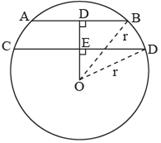QUESTION: 16

The shortest distance of the point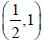from the curve y = |x – 1| + |x + 1| is

(2017)

Solution: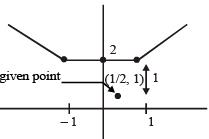shortest distance according graph = 1
y = |x – 1| + | x + 1 |
let x, – 1 ≤ x ≤ 1
y = – x + 1 + x + 1 ⇒ y = 2

QUESTION: 17

Let ABC be a right-angled isosceles triangle with hypotenuse BC. Let BQC be a semi-circle, away from A, with diameter BC. Let BPC be an arc of a circle centered at A and lying between BC and BQC. If AB has length 6 cm then the area, in sq cm, of the region enclosed by BPC and BQC is

(2017)

Solution: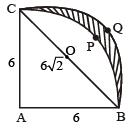Enclosed Area = Area of semi circle BQC with centre O – Area of Arc BPC with O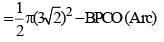...(i)
Now, Area of triangle ABC + BPCO (Arc) = Area of Quarter circle (ABPC)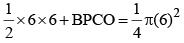Area of BPCO = 9π –18
Put the value in equation (i)
Enclosed Area =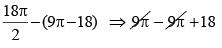= 18 sq cm

QUESTION: 18

From a triangle ABC with sides of lengths 40 ft, 25 ft and 35 ft, a triangular portion GBC is cut off where G is the centroid of ABC. The area, in sq ft, of the remaining portion of triangle ABC is

(2017)

Solution: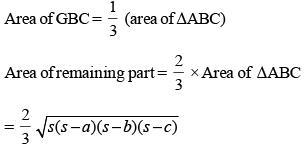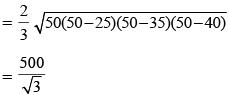QUESTION: 19

In the figure below, BD = 8 cm and DC = 6 cm. AE : ED = 3 : 4. If AF =12 cm, find AC (in cm).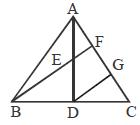(2016)

Solution:

Let G be a point on AC such that DG is parallel to BF.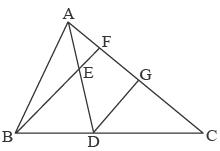Here, BD = 8 cm
DC = 6 cm and
AE : ED = 3 : 4
AF = 12 cm
AC = ?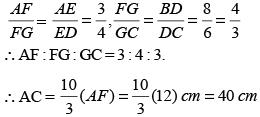QUESTION: 20

In the figure below, P, Q and R are points on a circle with centre O. The tangent to the circle at R intersects secant PQ at T. If ∠QRT = 55° and ∠QTR = 25°, find ∠POQ.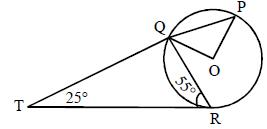(2016)

Solution: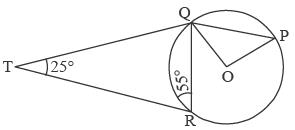In ΔQTR,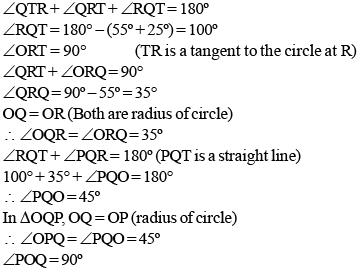QUESTION: 21

In the figure, O and O’ are the centres of the bigger and smaller circles respectively and small circle touches the square ABCD at the mid point of side AD. The radius of the bigger circle is equal to 15 cm and the side of the square ABCD is 18 cm. Find the radius of the smaller circle.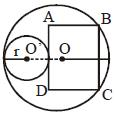(2015)

Solution: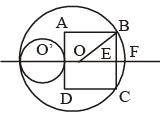Let the radius of the bigger circle be R and the smaller circle be r and the side of the square is 2a.
∴ OE = R – EF
= R – [2R – (2r + 2a)]
OE2 + EB2 = OB2
i.e  [2a +2r – R]2 +a2  = R2
a = 9 (∵ 2a = 18); R = 15
∴ (18 + 2r – 15)2 + 92 =152
∴ 2r + 3 = 12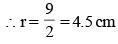Radius of smaller circle = 4.5 cm.

QUESTION: 22

ΔABC is a right angled triangle, with ∠B = 90o, AB = 20 cm and BC = 21cm. A circle with centre O is inscribed in triangle ABC. OD, OE and OF are perpendiculars drawn on the sides AB, BC and CA respectively. Find the ratio of the area of the quadrilateral FOEC to the area of the quadrilateral ADOF.

(2015)

Solution: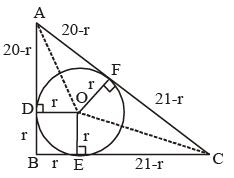∴  AB = 20
BC = 21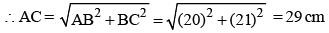and (20 – r) + (21 – r) = 29
or, 2r = 41 – 29
⇒ r = 6 cm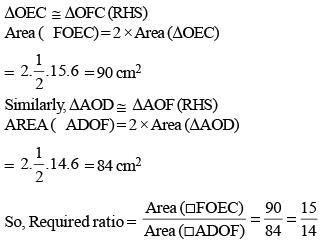QUESTION: 23

An isosceles right angled triangle with length of its equal sides being 30 cm, is rotated 180° about its centroid to form a new triangle. Find the area of the region common to the original and the new triangles.

(2015)

Solution: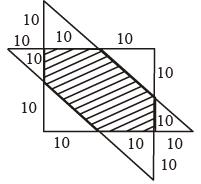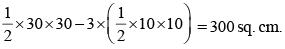QUESTION: 24

The line √3y = x is the radius of the circle, it meets the circle centred at origin O at point M(√3,1) If PQ is the tangent to the circle at M as shown, find the length of the PQ.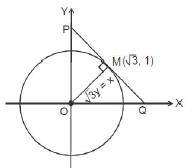(2015)

Solution:

PQ is perpendicular to line y =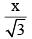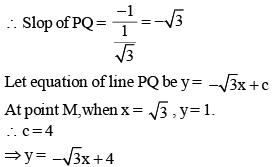∴ Co-ordinates of point Q =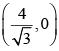and Co-ordinates of point P = (0, 4).
Hence,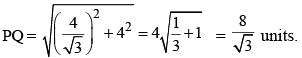QUESTION: 25

In the given figure, AB is the diameter of the circle with centre O. If ∠BOD = 15º, ∠EOA = 85º, then find the measure of ∠ECA.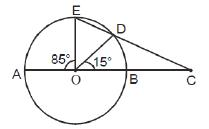(2015)

Solution:

Here, ∠EOQ = 85° and
∠BOD = 15°
∴ ∠EOD = 180° – (85° + 15°) = 80°
∴ In ∠OED
OE = OD (radius), ∠OED = ∠ODE = θ° (let)
∴ θ + θ + 80° = 180
⇒ 2θ° = 180 – 80 = 100°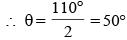∴ ∠OED = ∠ODE = 50°
In ∠EOC,
∠EOC = 80° + 15° = 95°
and ∠OEC = 50°
∴ ∠ECA =180° – (95° + 50°) = 35°

QUESTION: 26

The bisector of ÐBAC of Δ ABC cuts BC at D and the circumcircle of the triangle at E. If DE = 3 cm, AC = 4 cm and AD = 5 cm, then the length of AB is

(2014)

Solution: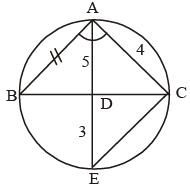The figure would be as shown above.
Join EC.
and ∠ABD = y°
⇒ ∠DAC = x°
and ∠AEC = y°
In ΔABD and ΔAEC,
∠ABD = ∠AEC
∴ ΔABD ~ ∠AEC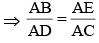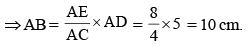QUESTION: 27

Let S be an arbitrary point on the side PQ of an acute-angled D PQR. Let T be the point of intersection of QR and the straight line PT drawn parallel to SR through P. Let U be the point of intersection of PR and the straight line QU drawn parallel to SR through Q. If PT = a units and QU = b units, then the length of SR is

(2014)

Solution: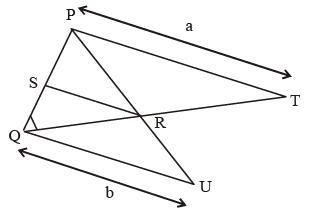Given that PT||SR|| QU
PT = a units, QU = b units.
ΔPTQ and ΔSRQ are similar.
∴ We have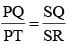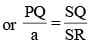...(i)
ΔUQP and ΔRSP are similar.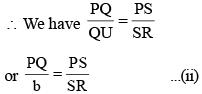Combining (i) and (ii)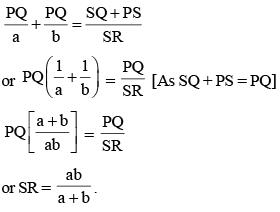QUESTION: 28

ABCD is a rectangle with points E and F lying on sides AB and CD respectively. If the area of quadrilateral AEFD equals the area of quadrilateral CBEF, then which of the following statements is necessarily false with respect to the rectangle ABCD?

(2014)

Solution:

Option (a) – The length of AE is always equal to the length of CF because E and F is midpoint of side AB and CD.
So, statement – I is always true.
Option (b) : the Length of BC is equal to length of EF so statement II is always False.
Option (c) : Length of AE is equal to the length of DF because of EF is parallel to BC
Option (d) : ∠AEF = ∠EFC = 90°
So statement III is also true.

QUESTION: 29

AC is a chord of a circle whose centre is at O. If B is any point on the arc AC and ∠OCA = 20°, then the magnitude of ∠ABC is

(2014)

Solution:

Here, Two cases are possible
Case I : When B lies in greater arc AC.
Then,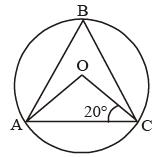∠OAC = ∠OCA = 20°
⇒ ∠AOC = 140°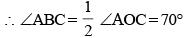Case II : When B lies in smaller arc AC.
Then,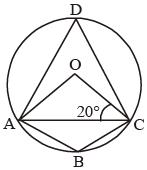Let D be a point O is the greater arc AC.
∠OAC =∠OCA = 20°
⇒ ∠AOC = 140°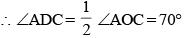∴ ∠ABC = 180° – 70° = 110°

QUESTION: 30

E is a point on the side AB of a rectangle ABCD, the adjacent sides of which are in the ratio 2 : 1. If ∠AED = ∠DEC, then what is the measure of ∠AED?

(2013)

Solution:

Let the two sides of the rectangle be x and 2x and ∠AED be θ.
∴ ∠DEC = θ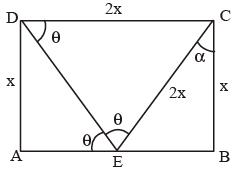∴ ∠CEB = 180 – 2θ
Also, ∠EDC = ∠AED = θ (AB is parallel to CD.) in ΔDEC,
∠DEC = ∠EDC ⇒ CD = EC = 2x
In ΔBCE,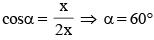Now, in ΔBCE
2θ = 90° + 60° (Exterior angle is equal to the sum of opposite interior angles.)
⇒ θ = 75°